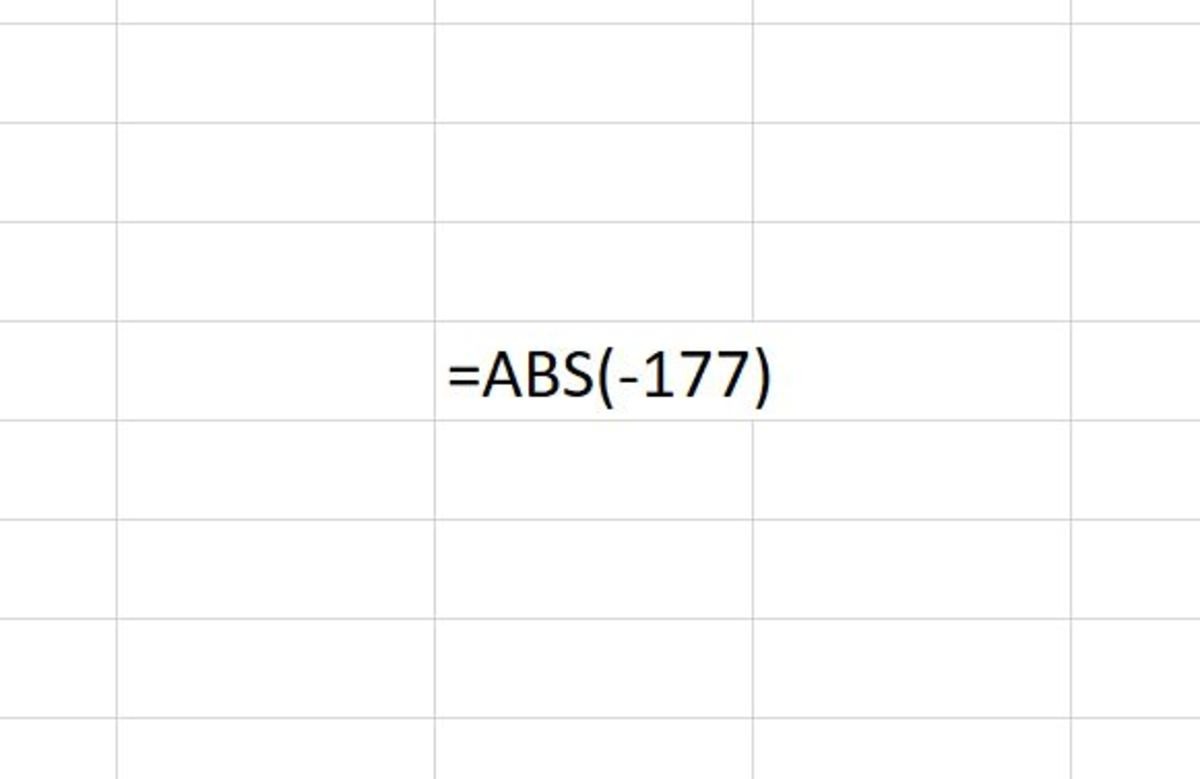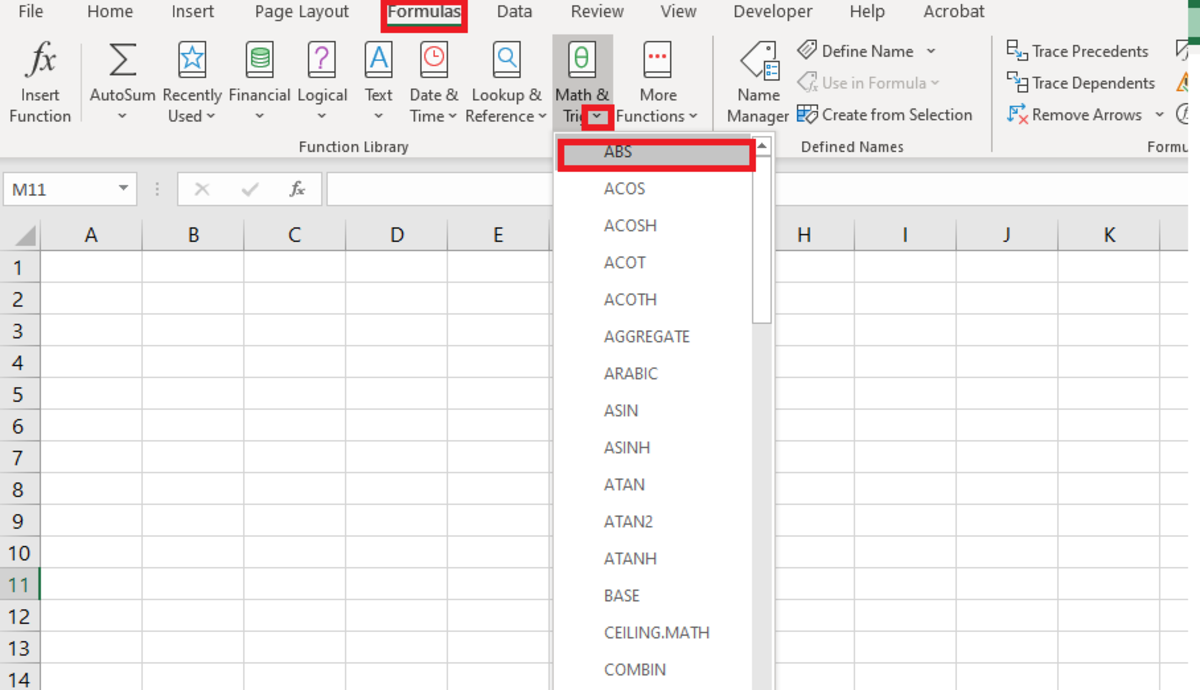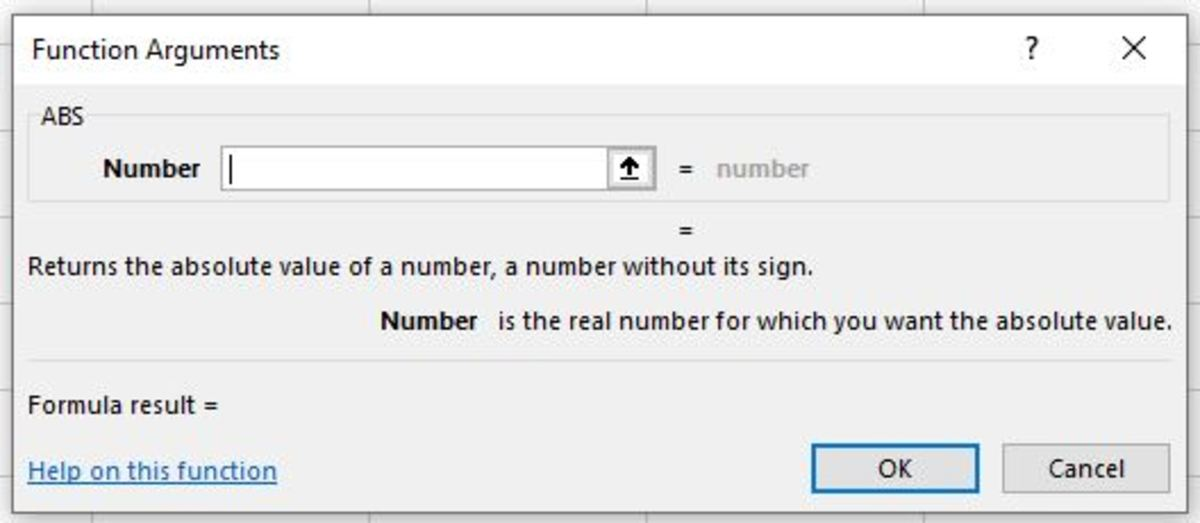# How to Use the ABS Function in Excel

Joshua is a graduate student at the USF. He has interests in business technology, analytics, finance, and lean six sigma.The ABS function appears above as it would when typed into an Excel worksheet cell. After this function is entered in the cell it would return a positive 177. Created by Joshua Crowder

## How the ABS Function Works

The absolute value function (ABS Function) allows an Excel user to return the absolute value of a number. So, if the ABS function is used with a negative number, a positive number would be returned. If the ABS function is used on a positive number, a positive number would also be returned. Additionally, it can be added to other calculations to find the absolute value of part of the calculation or the final result.

## ABS Function Syntax for Excel Worksheets

For this function to work in an Excel worksheet, it needs to be used with a real number (or reference a real number). The function can work in any of the three scenarios described below:

=ABS(5)

In this case, the absolute value of 5 will be returned. The result that will remain in the cell will be a 5. This shows that when a positive number is used with the ABS functions the same positive number will be returned.

=ABS(-5)

In this case, the absolute value of -5 will be returned. The result that will remain in the cell will be 5. Any negative numbers used with this function will become positive numbers.

=ABS(A1)

In this case, the absolute value of the number that is located in cell A1 will be returned. Additionally, cell references that have arithmetic (e.g. A1+B2) will also result in a positive number.

=ABS(5*-5)

In this case, the absolute value of the result will be returned. Any arithmetic calculated with this function will return a positive value. The answer that would be returned for this function would be 25.

=44*ABS(5*-5)

Scroll to Continue

Here the absolute value will only provide an absolute value for part of a formula. It can be added to any formula to find the absolute value of any part of a formula. This this situation might be used with some type of interval calculation.

## Entering the Formula Into a Worksheet

Whether the function is being used with a reference to another cell or as a number, it needs to be added to a cell in the form of a formula like the examples previously discussed. To create a formula, a cell needs to be clicked, and "=ABS(" needs to be typed in that cell or in the formula bar under the ribbon. After the open parenthesis, a cell reference, arithmetic with cell references, a number, or arithmetic with numbers needs to be added. This is then followed by a closing parenthesis. After the formula is completed, the enter button can be pressed to execute the formula.

## Inserting the ABS Function

The ABS function can also be inserted into a worksheet. To do so, a cell is first selected. Next, the formulas tab can be clicked, followed by the selection of the "Math & Trig" button on the Excel ribbon. The ABS selection from the list is then chosen.

## Inserting the ABS Function From the Formulas TabTo insert the ABS function from the formula tab you must first navigate to Formulas→Math & Trig→ABS Created by Joshua Crowder

After the function argument window appears, a cell reference, arithmetic with cell references, a number, or arithmetic with numbers needs to be added to the number field. Next, Ok can be selected to return the absolute value to the cell that was selected.Inserting the ABS function from the formula tabs gives you the advantage of not having to type the formula. The only data that needs to be entered into the functions arguments window is the numbers or cell references. Created by Joshua Crowder

## How to Use the Absolute Value Function in Excel

Microsoft. (n.d.). ABS function. Retrieved January 4, 2020, from https://support.office.com/en-us/article/abs-function-3420200f-5628-4e8c-99da-c99d7c87713c.

Crowder, J. (2020, January 4). How to Use the Absolute Value Function in Excel. Retrieved January 4, 2020, from https://youtu.be/gctQJ0rhm30.